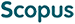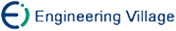韩爱丽, 朱大铭. 基于一种新的边权编码方案的中国邮递员问题的DNA计算模型[J]. 计算机研究与发展, 2007, 44(6): 1053-1062.
 引用本文: 韩爱丽, 朱大铭. 基于一种新的边权编码方案的中国邮递员问题的DNA计算模型[J]. 计算机研究与发展, 2007, 44(6): 1053-1062.Han Aili, Zhu Daming. DNA Computing Model Based on a New Scheme of Encoding Weight for Chinese Postman Problem[J]. Journal of Computer Research and Development, 2007, 44(6): 1053-1062.
 Citation: Han Aili, Zhu Daming. DNA Computing Model Based on a New Scheme of Encoding Weight for Chinese Postman Problem[J]. Journal of Computer Research and Development, 2007, 44(6): 1053-1062.## DNA Computing Model Based on a New Scheme of Encoding Weight for Chinese Postman Problem

• 摘要: 权编码方法是DNA计算中一个重要且有挑战性的问题.设计了一种新的用于表示赋权图中边权的DNA编码方案，给出了用该方案求解中国邮递员问题的DNA算法，并利用Markov链分析了DNA算法中生成各种路径的随机过程.对于任一赋权图G=(V,E)，首先通过边到点映射把它转换为广义边图G′=(V′,E′).图G的每条边e\-i被分别映射为图G′的一个顶点v′\-i. 若G中e\-i与e\-j邻接，则连接G′中v′\-i和v′\-j. 若G中v\-i为奇顶点，则在与v\-i关联的边对应的G′的顶点上添加自环.用于编码顶点v′\-i的DNA串s\-i的长度等于边e\-i的权值.用于编码边v′\-iv′\-j的DNA串s\-\ij\为s\-i的后半部分与s\-j的前半部分并置后的逆补.所提出的DNA编码方案具有易于编码、易于推广且错误率低的特点.该工作可提高DNA计算中表示和处理数值的能力，扩展DNA计算求解最优化问题的范围.

Abstract: Weight encoding method is an important but challenging issue in DNA computing. A new DNA encoding scheme to represent weights on a weighted graph is devised inthis paper and a corresponding DNA algorithm for the Chinese postman problem is proposed using the encoding scheme. The random procedure of generating various paths in the DNA algorithm is analyzed by means of Markov chain. For any weighted graph G=(V,E), it is firstly converted into its general edge graph G′=(V′,E′) through mapping from edges to vertices. Each edge e\-i in G is mapped as a vertex v′\-i in G′, and the vertices v′\-i and v′\-j in G′ are linked if e\-i and e\-j are adjacent in G. If v\-i in G is an odd degree vertex, the self-loops are added to the vertices in G′ which are mapped from the edges linked to v\-i. The length of DNA strand s\-i, which is used to encode vertex v′\-i, is equal to the value of weight on edge e\-i. The DNA strand s\-\ij\, which is used to encode edge v′\-iv′\-j, is the reverse complement of the second half of s\-i and the first half of s\-j. The proposed DNA encoding scheme has characteristics of easy encoding, easy generalizing and low error rate. This work improves the capability of representing and dealing with data and extends the range of solving numerical optimization problems in DNA computing./下载:  全尺寸图片 幻灯片
• 分享
• 用微信扫码二维码

分享至好友和朋友圈